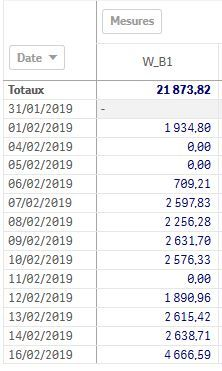# New to QlikView

Discussion board where members can get started with QlikView.

Announcements
Coming your way, the Qlik Data Revolution Virtual Summit. October 27-29. REGISTER
cancel
Showing results for
Did you mean:
HighlightedContributor

## Sum total with above not correct

Hi Everyone,

I need help for total result is not correct if someone can help Im begginer thanks

this my expression :

If(RowNo()=0 ,sum( aggr(FABS((Max(W_B1)))-above(Max(W_B1)),Date)),max(W_B1)-Above(max(W_B1)))/100

It should be get 24517,83 NOT 21873,82Labels (3)

• ### Total

1 Solution

Accepted Solutions
HighlightedMVP

Can you try this

```Sum(Aggr(
If(RowNo() = 0, Sum(Aggr(fabs((Max(W_B1))) -Above(Max(W_B1)), (Date, (NUMERIC)))), Max(W_B1) - Above(Max(W_B1)))/100
, (Date, (NUMERIC))))```
6 Replies
HighlightedMVP

May be try this

```Sum(Aggr(
If(RowNo() = 0, Sum(Aggr(fabs((Max(W_B1))) -Above(Max(W_B1)), Date)), Max(W_B1) - Above(Max(W_B1)))/100
, Date))```
HighlightedContributor

not work for me I get negative value.HighlightedMVP

Would you be able to share a sample to test this out?

HighlightedContributor

OKHighlightedMVP

Can you try this

```Sum(Aggr(
If(RowNo() = 0, Sum(Aggr(fabs((Max(W_B1))) -Above(Max(W_B1)), (Date, (NUMERIC)))), Max(W_B1) - Above(Max(W_B1)))/100
, (Date, (NUMERIC))))```Contributor

thanks a lot it's work for me